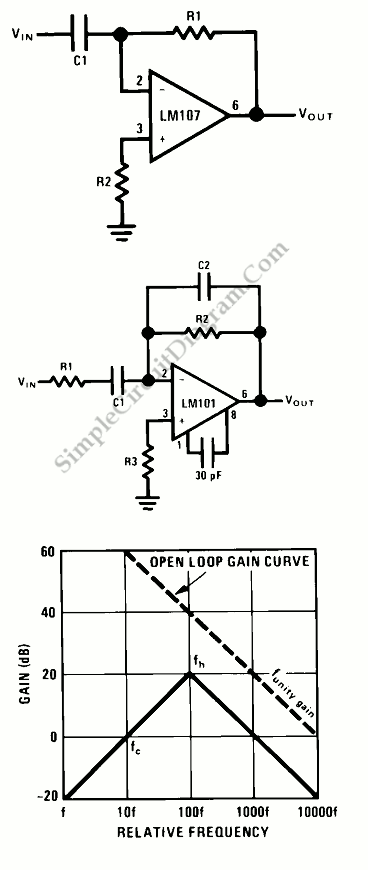# Op-Amp Circuit: Differentiator

This is a differentiator circuit. This circuit can be used to do differential operation. There are two differentiator, the are true differentiator and practical differentiator. The true differentiator is extremely susceptible to high frequency noise due to the increasing AC gain at the rate of 6 dB per octave. Here is the circuit of true differentiator, practical differentiator and Differentiator Frequency Response :The practical differentiator uses additional components, C2 and R1 to correct the noise problems and stability problems. To reduce amplifier noise and the effect of high frequency input, R1C1 form a 6 dB per octave roll-off network in the input network and a 6 dB per octave high frequency roll-off in the feedback network also formed by R2 and C2.

R1C1 is a RC low pass filter that contributes 90° phase shift to the loop. If we use just R1C1, it may cause stability problems so we can add the R2C2. R1C1 and R2C2 give 90° phase lead to compensate the 90°phase lag of R2C1 and prevent loop instability. [Source: National Semiconductor Application Note]